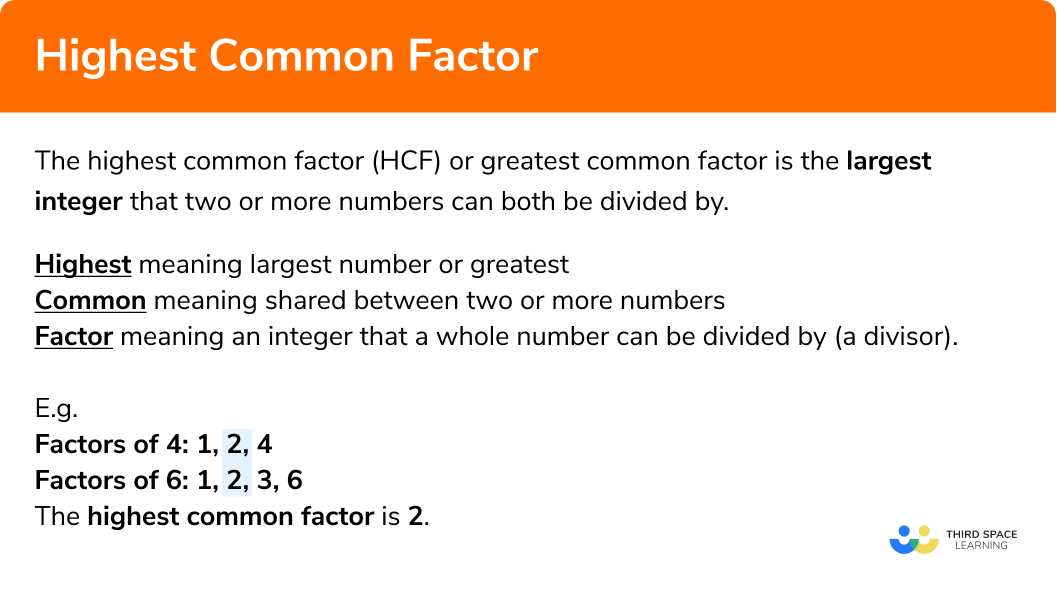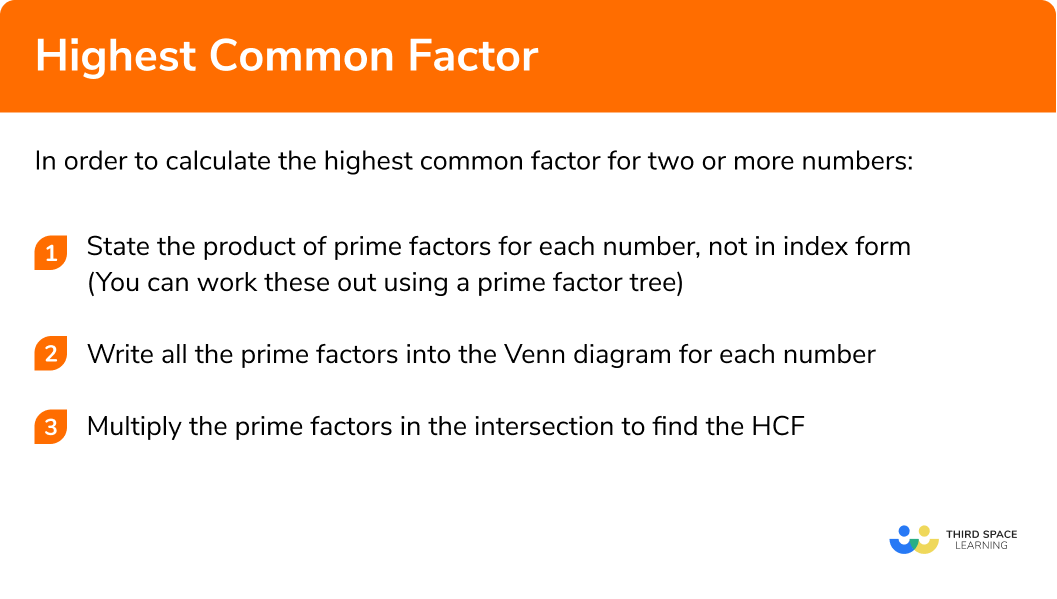# Highest Common Factor

Here we will learn about the highest common factor including how to calculate the highest common factor, use the highest common factor, and recognise when to calculate the highest common factor to answer complex worded problems.

There are also highest common factor worksheets based on Edexcel, AQA and OCR exam questions, along with further guidance on where to go next if you’re still stuck.

## What is the highest common factor?

The highest common factor (HCF) or greatest common factor is the largest integer that two or more numbers can be divided by.

Highest meaning largest number or greatest.
Common meaning shared between two or more numbers.
Factor meaning an integer that a whole number can be divided by (a divisor).

There are several interchangeable terms you should be aware of:

HCF represents the highest common factor, GCF represents the greatest common factor, and GCD represents the greatest common divisor. These are all the same!

E.g.
Find the HCF of 4, and 6.

Let’s start by writing the factors of 4 and 6,

We can see that the highest number that occurs in each list is 2,
hence for the numbers 4 and 6 the highest common factor is 2.

NOTE: 1 occurs in both lists so it is a common factor, but not the highest common factor.

### What is the highest common factor?### Highest common factors and prime factors

Calculating the highest common factor becomes more complicated for larger numbers as listing all the factors of each number is very time consuming.

To make it easier we can utilise the prime factors of the numbers.

The fundamental theorem of arithmetic states that each number greater than 1 is either a prime number or can be written by a product of primes.

This means that every number has a unique set of prime factors

We can use prime factors and a Venn diagram to calculate the highest common factor.
To do this we need to write out the prime factorisation of each number fully (without any powers) and then put the numbers into the Venn diagram by looking for pairs.

Here, we have the prime factors of 12 and 30.

• In the left circle are the prime factors of 12 (2, 2, and 3).
• In the right circle, we have the prime factors of 30 (2, 3, and 5).
• The dark orange section on the left represents the prime factors of 12 that are not prime factors of 30 (which is just the value 2).
• The light orange section on the right represents the prime factors of 30 that are not prime factors of 12 (which is just the value 5).
• The intersection represents the prime factors of 12 and 30.

Here, the prime factors of 2 and 3 occur for both 12 and 30 so their product is the highest common factor.

The highest common factor for 12 and 30 is 2 × 3 = 6.

### Why does the intersection contain the HCF?

The reason why the intersection contains the highest common factor is because there are no other common prime factors in this set of numbers, otherwise they would be in the intersection.

You must remember to write every factor out separately into the Venn diagram.

If you have more than one of the same prime factor (e.g. 12 = 2 × 2 × 3), we have to write each into the Venn diagram separately, otherwise the prime factorisation is not correct.

## How to find the highest common factor

In order to calculate the highest common factor for two or more numbers:

1. State the product of prime factors for each number, not in index form
(you can work these out using a prime factor tree)
2. Write all the prime factors into the Venn diagram for each number
3. Multiply the prime factors in the intersection to find the HCF

### Explain how to calculate the highest common factor for two or more numbers in 3 steps### Related lessons onfactors, multiples and primes

Highest common factor is part of our series of lessons to support revision on factors, multiples and primes. You may find it helpful to start with the main factors, multiples and primes lesson for a summary of what to expect, or use the step by step guides below for further detail on individual topics. Other lessons in this series include:

## Highest common factor examples

### Example 1: Two simple composite numbers

Calculate the highest common factor of 18 and 24.

1. State the product of prime factors for each number, not in index form

18 = 2 × 3 × 3

24 = 2 × 2 × 2 × 3

2Write all the prime factors into the Venn diagram for each number

3Multiply the prime factors in the intersection to find the HCF

HCF = 2 × 3 = 6

### Example 2: One composite number is a factor of the other

Calculate the highest common factor of 14 and 56.

14 = 2 × 7

56 = 2 × 2 × 2 × 7

HCF = 2 × 7 = 14

### Example 3: Calculate the prime factorisation first

Given that 90 = 2 × 32 × 5, calculate the highest common factor of 54 and 90.

To state the product of prime factors of 54, we use a factor tree:

54 = 2 × 3 × 3 × 3

90 = 2 × 3 × 3 × 5

HCF = 2 × 3 × 3 = 18

### Example 4: Three composite numbers

Calculate the highest common factor of 12, 20, and 32.

12 = 2 × 2 × 3

20 = 2 × 2 × 5

32 = 2 × 2 × 2 × 2 × 2

HCF = 2 × 2 = 4

### Example 5: Highest common factor of 1

Calculate the highest common factor of 35 and 72.

35 = 5 × 7

72 = 2 × 2 × 2 × 3 × 3

Here there are no prime factors in the intersection, so the highest common factor is equal to 1.

### Example 6: Worded problem

Sue sells apples in baskets. On Thursday, she will receive a delivery of 36 apples. On Friday, she will be delivered 42 apples. Sue wants to divide each crate equally into smaller baskets, so she has the same number of apples in each basket. What is the maximum number of apples she can put in each basket so that they contain the same quantity each day?

36 = 2 × 2 × 3 × 3

42 = 2 × 3 × 7

HCF = 2 × 3 = 6

So there will be 6 apples in each basket.

### Common misconceptions

• Calculating the Lowest Common Multiple (LCM) instead

A very common misconception is mixing up the highest common factor with the lowest common multiple.
Remember:
Factors are composite numbers that are split into smaller factors
Multiples are composite numbers that are multiplied to make larger multiples

• Incorrect evaluation of powers

It is possible to write prime factors into a Venn diagram with their associated exponent or power. This becomes an issue when the powers are not correctly interpreted.

Take for example the numbers 54 and 81.

54 = 2 × 33

81 = 34

This means that we have common factors of 3, but how many do we write in the intersection of the Venn diagram?
The correct answer is 3 as we have 3 lots of 3 for 54 and 81:

The remaining factor of 3 for 81 would be placed on the right hand blue circle and the remaining factor of 2 for 54 would be placed in the left hand yellow circle.

The Venn diagram would therefore look like this:

It is recommended that you write out each product of prime factors without using index form as this will make writing the numbers into the Venn diagram easier.

### Practice highest common factor questions

1. Calculate the highest common factor of 22 and 60 .

166011222= 2 \times 11 \\ 60= 2 \times 2 \times 3 \times 5HCF = 2

2. Calculate the highest common factor of 21 and 63 .

37216321=3 \times 7\\ 63 = 3 \times 3 \times 7\text{HCF }=7 \times 3=21

3. Calculate the highest common factor of 90 and 135 .

4515127090= 2 \times 3 \times 3 \times 5 \\ 135=3 \times 3 \times 3 \times 5\text{HCF }=3\times 3 \times 5=45

4. Calculate the highest common factor of 12 , 30 and 48 .

1223612=2 \times 2 \times 3\\ 30=2 \times 3 \times 5\\ 48=2 \times 2 \times 2 \times 2 \times 3\text{HCF }= 2 \times 3=6

5. Calculate the highest common factor of 21 and 58 .

013121821=3 \times 7\\ 58= 2 \times 29HCF=1

6. Josh is grouping 3 different coloured writing pens. He has 18 blue, 16 black, and 28 red. Each group must contain the same amount of each colour pen. How many groups can Josh make, so that every pen has been grouped?

2141008We need to calculate the HCF of  18, 16  and  28. \\
18=2 \times 3 \times 3\\ 16=2 \times 2 \times 2 \times 2\\ 28=2 \times 2 \times 7 \\HCF=2
Therefore Josh can make  2  groups of pens with the same number of each colour in each group.

### Highest common factor GCSE questions

1. The highest common factor of a and b is 5 . The lowest common multiple of a and b is 30 . State the values of a and b .

(4 marks)(1)

30 \div 5=6

(1)

 6= 2 \times 3

(1)2\times 5=10 \text{ and } 5 \times 3=15
  a=10  and  b = 15

(1)

(accept the alternative solution:  a=15  and  b=10 )

2.
(a) Calculate the highest common factor of the two numbers a=16 g^{2} h^{2} \text { and } b=28 g^{3} h

(b) Let g=3 and h=5
(i) Calculate the values of a and b
(ii) Hence or otherwise, calculate the value of the highest common factor when g=3 and h=5

(8 marks)

\text { a) }16 g^{2} h^{2}=2 \times 2 \times 2 \times 2 \times g \times g \times h \times h \text { or } 28g^{3}h=2 \times 2 \times 7 \times g \times g \times g \times h

(1)

HCF \text { of } 16 \text { and } 28=2 \times 2=4

(1)

HCF \text { of } g^{2} h^{2} \text { and } g^{3} h=g^{2} h

(1)

HCF \text { of } 16 g^{2} h^{2} \text { and } 28g^{3}h =4g^{2}h

(1)

\text { b)(i) } a = 16 \times 3 \times 3 \times 5 \times 5 = 3600

(1)

b = 28 \times 3 \times 3 \times 3 \times 5 = 3780

(1)

\text { b)(ii) } 4 \times 3 \times 3 \times 5

(1)

180

(1)

3. A school is conducting a research project. Year 11 classes will be split into small groups with the same proportion of students from each class.

• Class 11 R has 32 pupils
• Class 11 B has 28 pupils
• Class 11 G has 36 pupils.

What is the maximum number of groups possible?

(3 marks)

32=2 \times 2 \times 2 \times 2 \times 2 \text { or } 32=2^{5}

(1)

28=2 \times 2 \times 7 . \text { and } 36=2 \times 2 \times 3 \times 3

(1)

\mathrm {HCF} (32,28 \text { and } 36)=4

(1)

## Learning checklist

You have now learned how to:

• Use the concepts and vocabulary of prime numbers, factors (or divisors), multiples, common factors, common multiples, highest common factor, lowest common multiple, prime factorisation, including using product notation and the unique factorisation property

## Still stuck?

Prepare your KS4 students for maths GCSEs success with Third Space Learning. Weekly online one to one GCSE maths revision lessons delivered by expert maths tutors.

Find out more about our GCSE maths tuition programme.